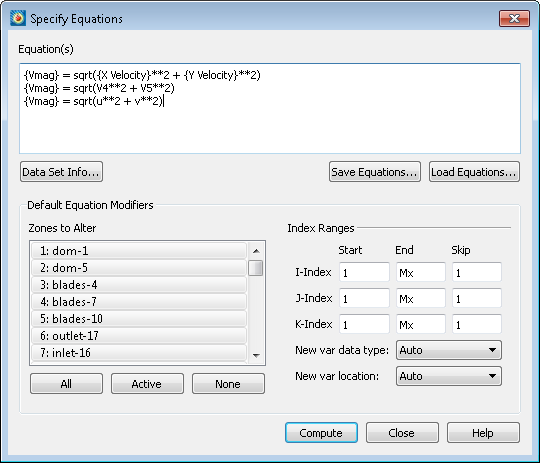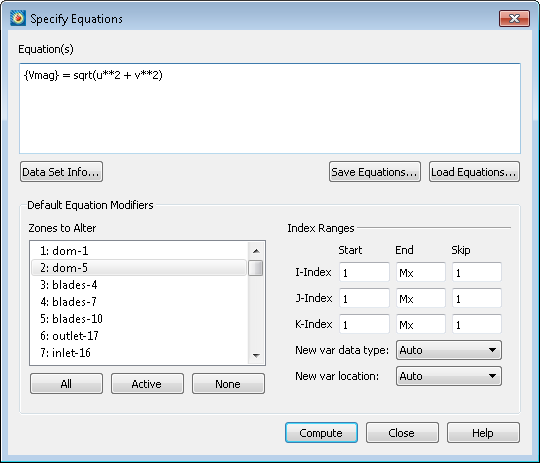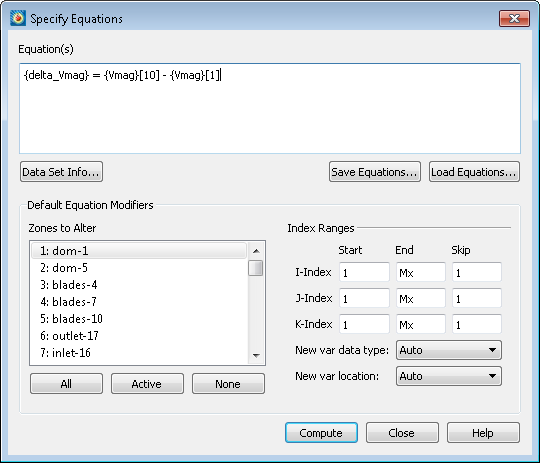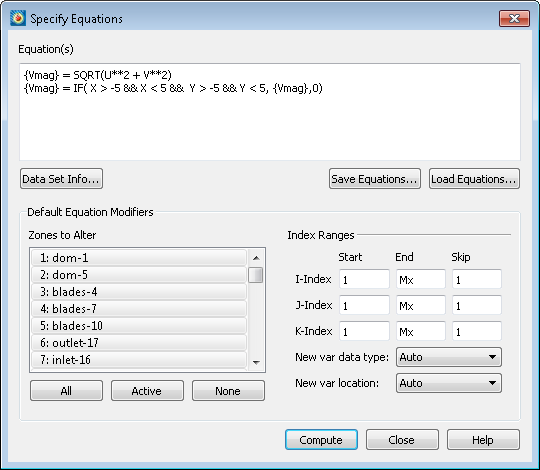# Calculating a New Variable

Creating a new variable or referencing a variable by name requires surrounding it in curly braces.

Variables on the right side of equations may be referenced by two other methods:  First, by the variable index. Second is to use variable assignment letter codes. In this example, we’re using ‘u’ and ‘v’ which represent the variables assigned to the U and V vector components in Plot -> Vector -> Variables dialog.  A complete list of variable assignment letter codes is available in Section 20 – 1.1, “Equation Syntax” in the User’s Manual.

Common mathematical functions such as SIN, COS, SQRT, and other common mathematical operands are available in the equation syntax. Notably, squared or second power is indicated by a double star. A full list of functions is located in Section 20 – 1.1, “Equation Syntax” in the User’s Manual.For our velocity magnitude calculation, all three of these equations will achieve the same result.

## Editing Specific Zones

If you only want to edit or create a variable for one zone, select the zone you wish to use in the “Zones to Alter” field.For this example, only the second zone will be edited and Vmag will be calculated using only its velocity components.

If you wanted to compare two zones, find two zones of identical grid structure and enter the following syntax:This will create a new variable called “delta_Vmag” which takes the difference of the tenth and first zone. The result will be applied only to the first zone due to the selection in “Zones to Alter”.

To see a full example of the bracket syntax, watch Comparing Grids in Tecplot 360.

## Using IF statements

Let’s say you only want to edit a specific portion of your dataset. You can specify these conditions with an IF statement. Let’s take our Vmag variable we have already created and make it 0 outside of a specific region:This equation reiterates our Vmag calculation and then adds our IF statement. This IF condition makes all of the Vmag values outside our bounding box, equal to zero. Our bounding box chosen in this situation is X from -5 to 5, and Y from -5 to 5.

If you would like to learn additional capabilities and examples of the Specify equations dialog, check out previous tutorial videos: Data Alter using If Conditions or Comparing a CFD solution with Experimental Data from the external flow series.